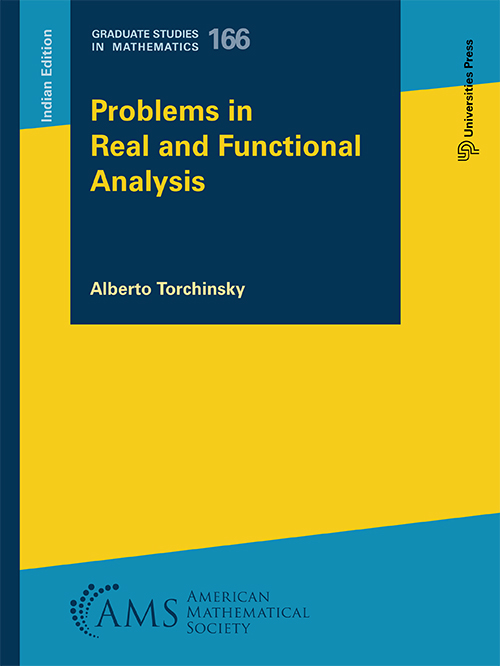## Problems in Real and Functional Analysis

#### Price: 1875.00

It is generally believed that solving problems is the most important part of the learning process in mathematics because it forces students to truly understand the definitions, comb through the theorems and proofs, and think at length about the mathematics. The purpose of this book is to complement the existing literature in introductory real and functional analysis at the graduate level with a variety of conceptual problems (1,457 in total), ranging from easily accessible to thought provoking, mixing the practical and the theoretical aspects of the subject. Problems are grouped into ten chapters covering the main topics usually taught in courses on real and functional analysis. Each of these chapters opens with a brief reader’s guide stating the needed definitions and basic results in the area and closes with a short description of the problems.

The Problem chapters are accompanied by Solution chapters, which include solutions to two-thirds of the problems. Students can expect the solutions to be written in a direct language that they can understand; usually the most “natural” rather than the most elegant solution is presented.

#### Contributors (Author(s), Editor(s), Translator(s), Illustrator(s) etc.)

Alberto Torchinsky, Indiana University, Bloomington, IN

Preface
Part 1. Problems
Chapter 1. Set Theory and Metric Spaces
Problems
Chapter 2. Measures
Problems
Chapter 3. Lebesgue Measure
Problems
Chapter 4. Measurable and Integrable Functions
Problems
Chapter 5. Lp Spaces
Problems
Chapter 6. Sequences of Functions
Problems
Chapter 7. Product Measures
Problems
Chapter 8. Normed Linear Spaces. Functionals
Problems
Chapter 9. Normed Linear Spaces. Linear Operators
Problems
Chapter 10. Hilbert Spaces
Problems
Part 2. Solutions
Chapter 11. Set Theory and Metric Spaces
Solutions
Chapter 12. Measures
Solutions
Chapter 13. Lebesgue Measure
Solutions
Chapter 14. Measurable and Integrable Functions
Solutions
Chapter 15. Lp Spaces
Solutions
Chapter 16. Sequences of Functions
Solutions
Chapter 17. Product Measures
Solutions
Chapter 18. Normed Linear Spaces. Functionals
Solutions
Chapter 19. Normed Linear Spaces. Linear Operators
Solutions
Chapter 20. Hilbert Spaces
Solutions

Index

`